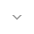belongs to Maven artifact com.android.support:support-compat:28.0.0-alpha1

# Pair

``` public class Pair ```
``` extends Object ``` ``` ```

 java.lang.Object ↳ android.support.v4.util.Pair

Container to ease passing around a tuple of two objects. This object provides a sensible implementation of equals(), returning true if equals() is true on each of the contained objects.

## Summary

### Fields

``` public final F``` `first`

``` public final S``` `second`

### Public constructors

``` Pair(F first, S second) ```

Constructor for a Pair.

### Public methods

``` static <A, B> Pair<A, B>``` ``` create(A a, B b) ```

Convenience method for creating an appropriately typed pair.

``` boolean``` ``` equals(Object o) ```

Checks the two objects for equality by delegating to their respective `equals(Object)` methods.

``` int``` ``` hashCode() ```

Compute a hash code using the hash codes of the underlying objects

``` String``` ``` toString() ```

### Inherited methodsFrom class ``` java.lang.Object ```

## Fields

### first

`F first`

### second

`S second`

## Public constructors

### Pair

```Pair (F first,
S second)```

Constructor for a Pair.

Parameters
`first` `F`: the first object in the Pair

`second` `S`: the second object in the pair

## Public methods

### create

```Pair<A, B> create (A a,
B b)```

Convenience method for creating an appropriately typed pair.

Parameters
`a` `A`: the first object in the Pair

`b` `B`: the second object in the pair

Returns
`Pair<A, B>` a Pair that is templatized with the types of a and b

### equals

`boolean equals (Object o)`

Checks the two objects for